### Home > CALC > Chapter 7 > Lesson 7.1.4 > Problem7-46

7-46.
1. Consider the curve formed by the equation xy2x3y = 12. Homework Help ✎

1. Show that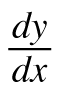=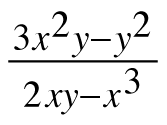.

2. Find the x-coordinate of each point on the curve where the tangent line is vertical.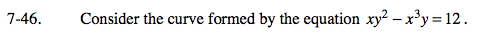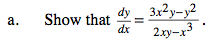Use implicit differentiation.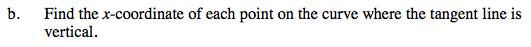A tangent line will be vertical where the denominator of the derivative = 0.

Since the denominator has both x and y values, there will be infinitely many ways to make the denominator equal to 0.
But only some (or one) of those ways fit the given curve.